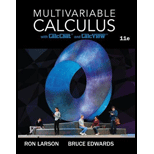# Motion of a Spring In Exercises 31-34, use the differential equation w g y ' ' ( t ) + b y ' ( t ) + k y ( t ) = w g F ( t ) which models the oscillating motion of an object on the end of a spring (see figure). In the equation, y is the displacement from equilibrium (positive direction is downward), measured in feet, t is time in seconds, w is the weight of the object, g is the acceleration due to gravity, b is the magnitude of the resistance to the motion, k is the spring constant from Hooke’s Law, and F ( t ) is the acceleration imposed on the system. Find the displacement y as a function of time t for the oscillating motion described subject to the initial conditions. Use a graphing utility to graph the displacement function. w = 2 , g = 32 , b = 1 , k = 4 , F ( t ) = 4 sin 8 t y ( 0 ) = 1 4 , y ' ( 0 ) = − 3### Multivariable Calculus

11th Edition
Ron Larson + 1 other
Publisher: Cengage Learning
ISBN: 9781337275378

#### Solutions

Chapter
Section### Multivariable Calculus

11th Edition
Ron Larson + 1 other
Publisher: Cengage Learning
ISBN: 9781337275378
Chapter 16.3, Problem 33E
Textbook Problem
1 views

## Motion of a Spring In Exercises 31-34, use the differential equation w g y ' ' ( t ) + b y ' ( t ) + k y ( t ) = w g F ( t ) which models the oscillating motion of an object on the end of a spring (see figure). In the equation, y is the displacement from equilibrium (positive direction is downward), measured in feet, t is time in seconds, w is the weight of the object, g is the acceleration due to gravity, b is the magnitude of the resistance to the motion, k is the spring constant from Hooke’s Law, and F(t) is the acceleration imposed on the system. Find the displacement y as a function of time t for the oscillating motion described subject to the initial conditions. Use a graphing utility to graph the displacement function. w = 2 , g = 32 , b = 1 , k = 4 , F ( t ) = 4 sin 8 t y ( 0 ) = 1 4 ,   y ' ( 0 ) = − 3

To determine

To calculate: The displacement y as a function of time t for the oscillating motion described subject to initial condition. Also graph the displacement function using graphing utility. The given differential equation is wgy''(t)+by'(t)+ky(t)=wgF(t) where w=2,g=32,b=1,k=4,F(t)=4sin8t.

### Explanation of Solution

Given information:

The given differential equation is wgy''(t)+by'(t)+ky(t)=wgF(t) where w=2,g=32,b=1,k=4,F(t)=4sin8t and assume that y(0)=14and y'(0)=3.

Concept Used:

If yp is the particular solution of the differential equation y''+ay'+by=F(x) and yh is the general solution, then y=yh+yp is the general solution of the non homogeneous equation.

Calculation:

Consider the given differential equation is wgy''(t)+by'(t)+ky(t)=wgF(t).

The coefficients of the differential terms are,

wg=232=116b=1k=4F(t)=4sin8t

Now putting these in the differential equation

116y''(t)+1×y'(t)+4×y(t)=116×4sin8t116y''(t)+y'(t)+4×y(t)=14sin8ty''(t)+16y'(t)+64×y(t)=4sin8t

The characteristics equation is m2+16m+64=0.

First, we have to find the solution of the characteristics equation m2+16m+64=0.

m2+16m+64=0(m+8)2=0m=8

Therefore,

yh=(C1+C2t)e8t

Let yp be generalized form of 14sin8t

### Still sussing out bartleby?

Check out a sample textbook solution.

See a sample solution

#### The Solution to Your Study Problems

Bartleby provides explanations to thousands of textbook problems written by our experts, many with advanced degrees!

Get Started

Find more solutions based on key concepts
For , which vector, a, b, c, or d, best represents F(1, 1)? a b c d

Study Guide for Stewart's Multivariable Calculus, 8th

Solve the equations in Exercises 112 for x (mentally, if possible). 34x+1=0

Finite Mathematics and Applied Calculus (MindTap Course List)

Evaluate . e + 1

Study Guide for Stewart's Single Variable Calculus: Early Transcendentals, 8th

In Exercises 5-8, plot the graph of the function f in an appropriate viewing window. (Note: The answer is not u...

Applied Calculus for the Managerial, Life, and Social Sciences: A Brief Approach

Perform the indicated operation: (5x+3)(3x4)

Elementary Technical Mathematics

In working further with the problem of exercise 4, statisticians suggested the use of the following curvilinear...

Modern Business Statistics with Microsoft Office Excel (with XLSTAT Education Edition Printed Access Card) (MindTap Course List)

A permutation of a set of elements is_________.

Discrete Mathematics With Applications

Finding Windows and Making Graphs: In Exercises S-11 through S-30, find an appropriate window setup and produce...

Functions and Change: A Modeling Approach to College Algebra (MindTap Course List)

In Problems 1926 solve the given differential equation. 21. (6x+1)y2dydx+3x2+2y3=0

A First Course in Differential Equations with Modeling Applications (MindTap Course List)